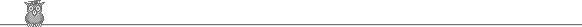### 16th APMO 2004Problem 4

Show that [(n-1)!/(n2+n)] is even for any positive integer n.

Solution

Thanks to Juan Ignacio Restrepo

For n = 1, 2, 3, 4, 5 we have [(n-1)!/(n2+n)] = 0, which is even. So assume n ≥ 6.

If n and n+1 are composite, then they must divide (n-1)!. They are coprime, so their product must divide (n-1)!. Note that just one of n, n+1 is even. For m ≥ 6, (m-2)! is divisible by more powers of 2 than m, so (n-1)!/(n2+n) is even. It remains to consider the two cases n+1 = p, a prime, and n = p a prime.

If n+1 = p, then p-1 is composite, so p-1 divides (p-2)!. Let k = (p-2)!/(p-1). By Wilson's theorem we have (p-2)! = 1 mod p, so k(p-1) = 1 mod p and hence k = -1 mod p. So (k+1)/p is an integer. But k is even, so k+1 is odd and hence (k+1)/p is odd. Now [k/p] = (k+1)/p - 1, so [k/p] = [(n-1)!/(n2+n)] is even.

If n = p, then k = (p-1)!/(p+1) is an even integer, so k+1 is an odd integer. By Wilson's theorem, k(p+1) = -1 mod p, so (k+1)/p is an integer and hence an odd integer. Hence [(n-1)!/(n2+n)] = [k/p] = (k+1)/p - 1 is even.

Wilson's theorem states that p is prime iff (p-1)! = -1 mod p. If p is composite, then it has a factor ≤ p-1, which divides (p-1)! and so does not divide (p-1)! + 1. Hence (p-1)! ≠ -1 mod p. Now suppose p is prime. If p = 2, then (p-1)! = 1 = -1 mod 2. So assume p is odd. Note first that if a2 = 1 mod p and 0 < a < p, then a = 1 or p-1. For p divides (a+1)(a-1) and so it divides either a+1, giving a = p-1, or it divides a-1, giving a = 1. Now for each 0 < a < p, there is a unique a' such that aa' = 1 mod p. We have just shown that a = a' iff a = 1 or p-1. So we can divide 2, 3, ... , p-2 into pairs with the product of each pair being 1 mod p. Hence (p-2)! = 1 mod p, as required.

On the powers of 2, note that 2, 22, 23, ... , 2k-1 < 2k and their product is 2k(k-1)/2. If 2k < n < 2k+1, then n is divisible by at most 2k-1. So for n ≥ 16, for example, (n-8)!/n is even. For n = 8, 9, ... , 15, (n-2)! is divisible by 2·4·6 and n is divisible by at most 8, so (n-2)!/n is even. Finally, we can easily check n = 6, 7.